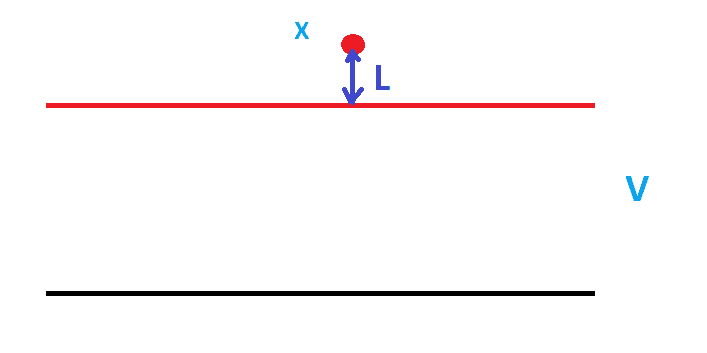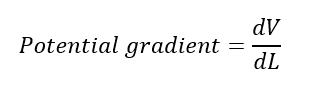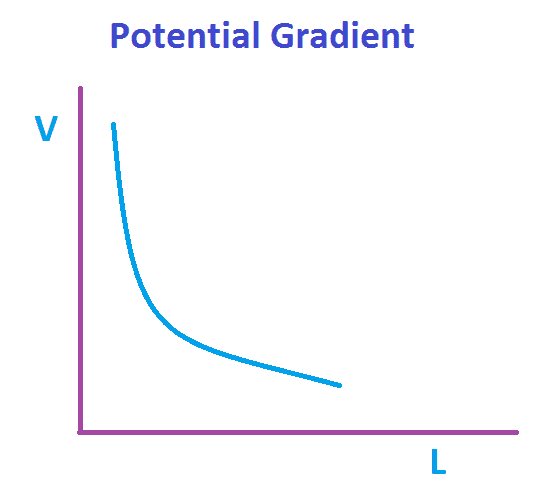# What is Potential Gradient in Electrical, Use of Potential Gradient Calculation

Potential gradient is nothing but the rate of change of electric potential with respect to displacement. It is also called as dielectric stress or voltage stress. Consider an object X is placed near to the high voltage line and L is the distance between the object X and the high voltage conductor,Then the Potential gradient formula can be expressed asUnit of the Potential gradient is Volt/meter typically V/m

Refer the graph between the Potential gradient and distanceWhen the distance of the object is less or near to the high voltage conductor the potential gradient is high, when you increase the distance the same decreases. At the same time when you increase the voltage the potential gradient also increases.